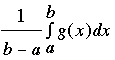SEARCH HOMEMath Central Quandaries & QueriesQuestion from Tom, a student: For the function x/3x-1 find the average rate of change between the interval x=1 and x=5?Hi Tom,

The average of a function g(x) from a to b isLet f(x) = x/(3x - 1) then the rate of change of f(x) is given by f '(x) thus you want the average of f '(x) from 1 to 5.

Look carefully at what you have to do. This problem is much easier than it seems.

Harley

Tom wrote back

I get the answer: (5/14) - (1/2) / (4) which = -1/28 but this is wrong.
I thought I was finding the slope of the secant line between x=1 x=5 for the function, hence the average rate of change.
Can you explain what i'm doing wrong and give me a correct answer? Thankyou

Hi again Tom,

I got the same numerical answer as you, -1/128 by using the technique in my earlier response. Can you tell me why you say "this is wrong"? Do you know what the "correct answer" is supposed to be?

HarleyMath Central is supported by the University of Regina and The Pacific Institute for the Mathematical Sciences.